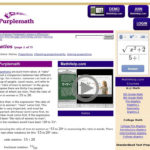# Tag Archives: angles & pythagorean theorem#### Ratios

Oktober 13th, 2017

This set of instructional materials and problems helps students understand ratios and proportions, starting with a simple review and progressing into the more advanced territory of similar triangles#### A Geometric Proof of the Pythagorean Theorem

Oktober 13th, 2017

Students will see an animated presentation of the proof of the Pythagorean Theorem. This animated PowerPoint presentation uses shearing and the invariance of the area of triangles with congruent bases and heights to show a step-by-step geometric proof of the Pythagorean Theorem.